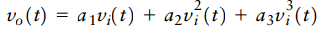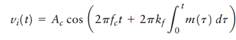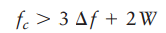Create an Account

Home / Questions / In this problem we explore how the use of FM can overcome nonlinear distortion Consider a ...

In this problem we explore how the use of FM can overcome nonlinear distortion Consider a memoryless channel characterized by the nonlinear input output relationship

In this problem, we explore how the use of FM can overcome nonlinear distortion. Consider a memoryless channel characterized by the nonlinear input–output relationship:where vi(t) is the input and υ0(t) is the output; α1, α2, and α3, are fixed coefficients. The input is defined by the frequency-modulated signalThe message bandwidth is denoted by W, and the frequency deviation of the FM signal is ∆f.

(a) Evaluate the output υ0(t).

(b) Using the generalized Carson rule, show that if the carrier frequency satisfies the conditionthen the effect of nonlinear distortion can be removed by band-pass filtering.

(c) Specify the mid-band frequency and bandwidth of the filter in part (b).

Jul 31 2020 View more View LessSubscribe To Get Solution